# Machine Learning From Scratch## Installation

Install spkit `pip install spkit`

``````from spkit.ml import LR, NaiveBayes, ClassificationTree, RegressionTree
``````

(Few visualisations are limited to 2D data only, others can be used for any dimenntions)

## 1. Logistic Regression

### Code ans examples are here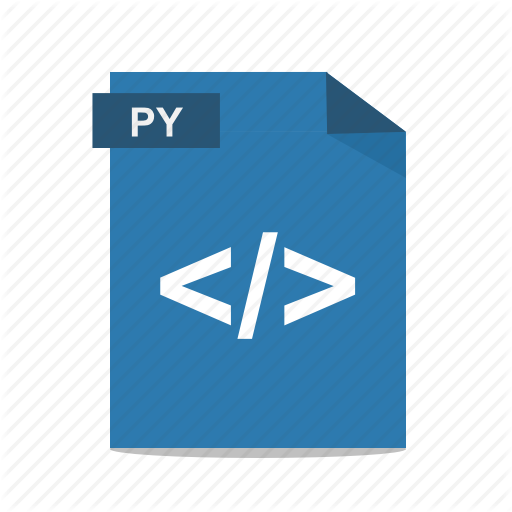``````#from LogisticRegression import LR # given code

##NOW USE spkit library (pip intall spkit)
from spkit.ml import LR
clf = LR(X,y,alpha=0.003,polyfit=True,degree=5,lambd=2)
fig=plt.figure(figsize=(8,4))
gs=GridSpec(1,2)

for i in range(100):
Clf.fit(X,y,itr=10)
ax1.clear()
Clf.Bplot(ax1,hardbound=False)
ax2.clear()
Clf.LCurvePlot(ax2)
fig.canvas.draw()
plt.pause(0.001)

clf.predict(X)
W,b =clf.getWeight()

``````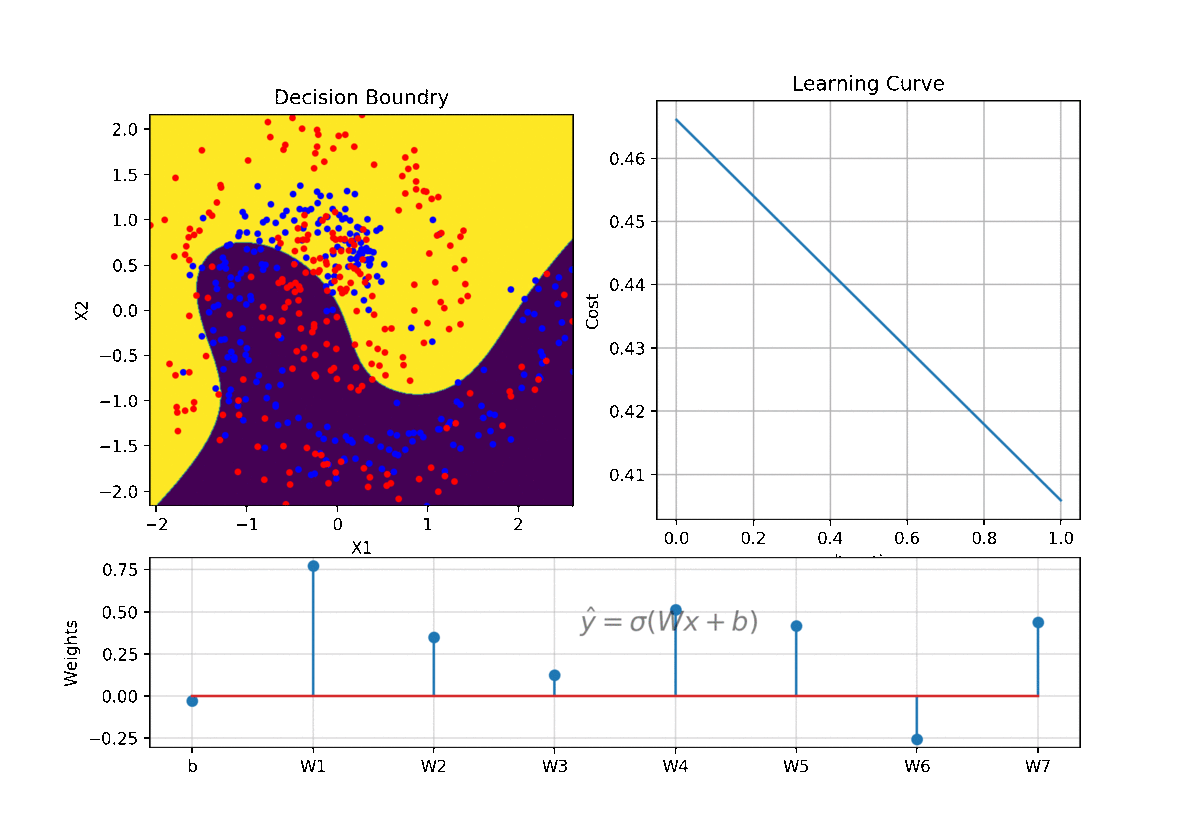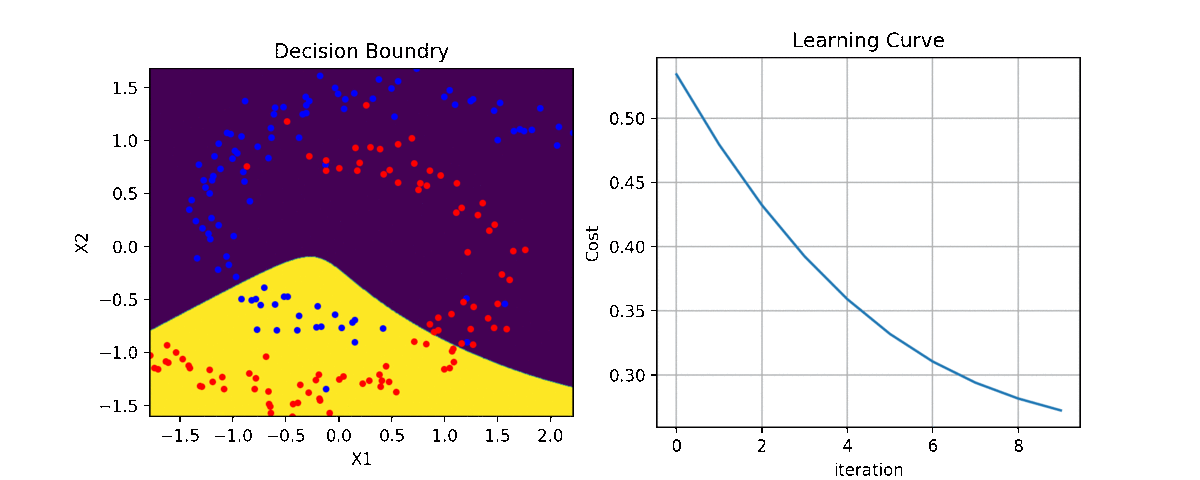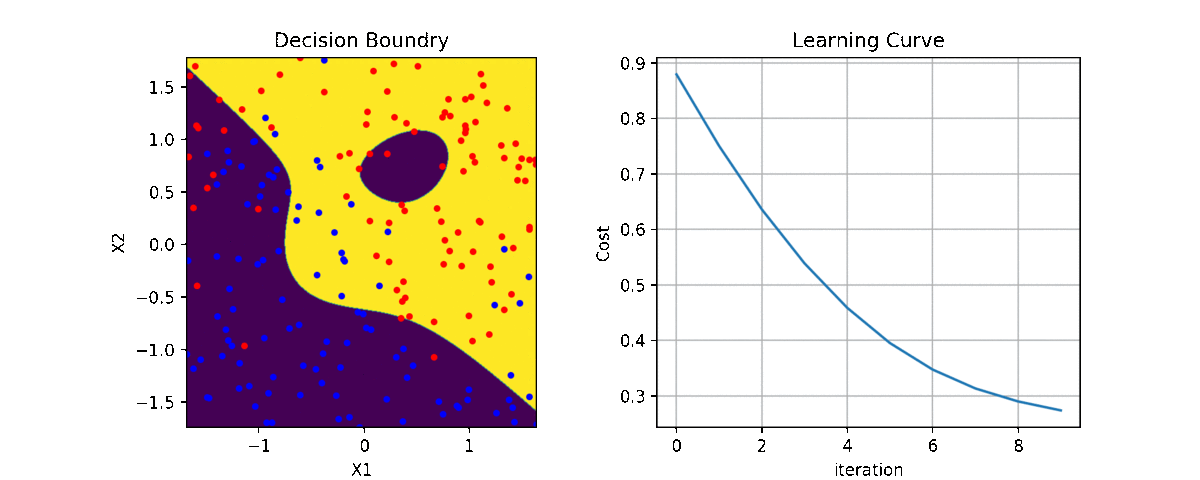## 2. Deep Neural Network - Deeplearning

### Code and examples are here

#### Full detail of implementation and use of code is describe here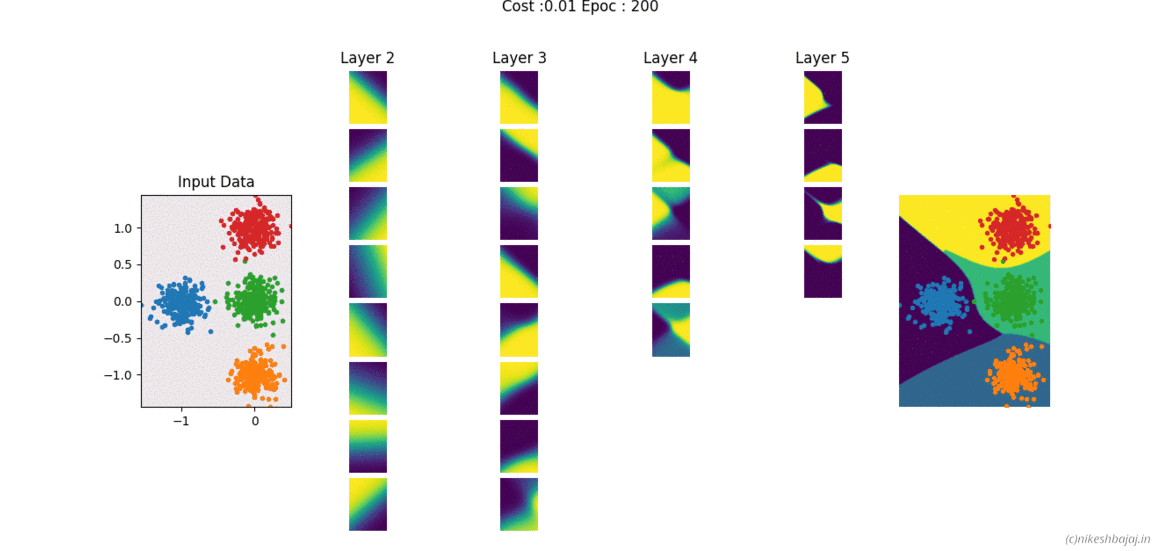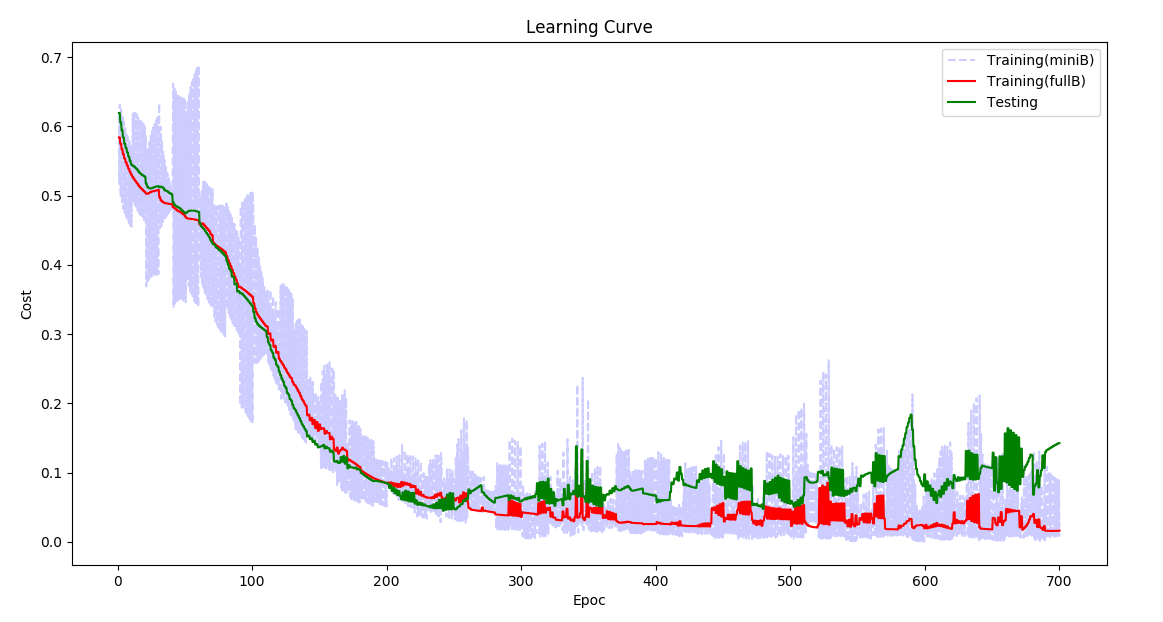## 3. Neural Network (simple structure-fully connected) with any number of layers (Matlab/Octave)

### Code and examples here

Network can be created and trained as for example

``````W= NeuralNet(X,y,HL,Iterations,alpha,verbose);

% For 2 hidden layers with 5 and 3 neurons, 500 iteration and 0.1 alpha(learning rate)
% input and output layers are chosen according to data X,y provided

W= NeuralNet(X,y,[5,3],500,0.1,1);

% for 8 hidden layers
W= NeuralNet(X,y,[15,10,10,10,5,5,5,3],100,0.1,1);

returns weights W of each layer

``````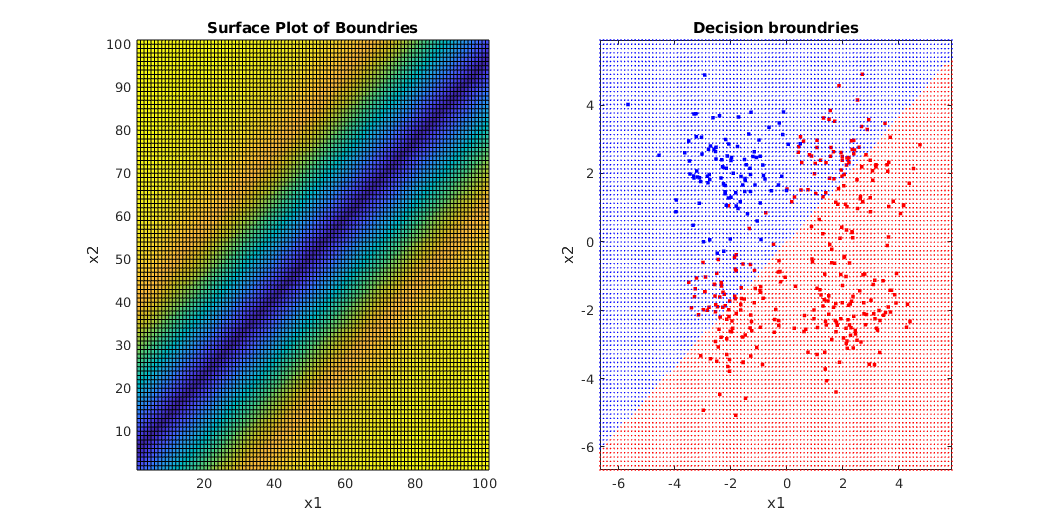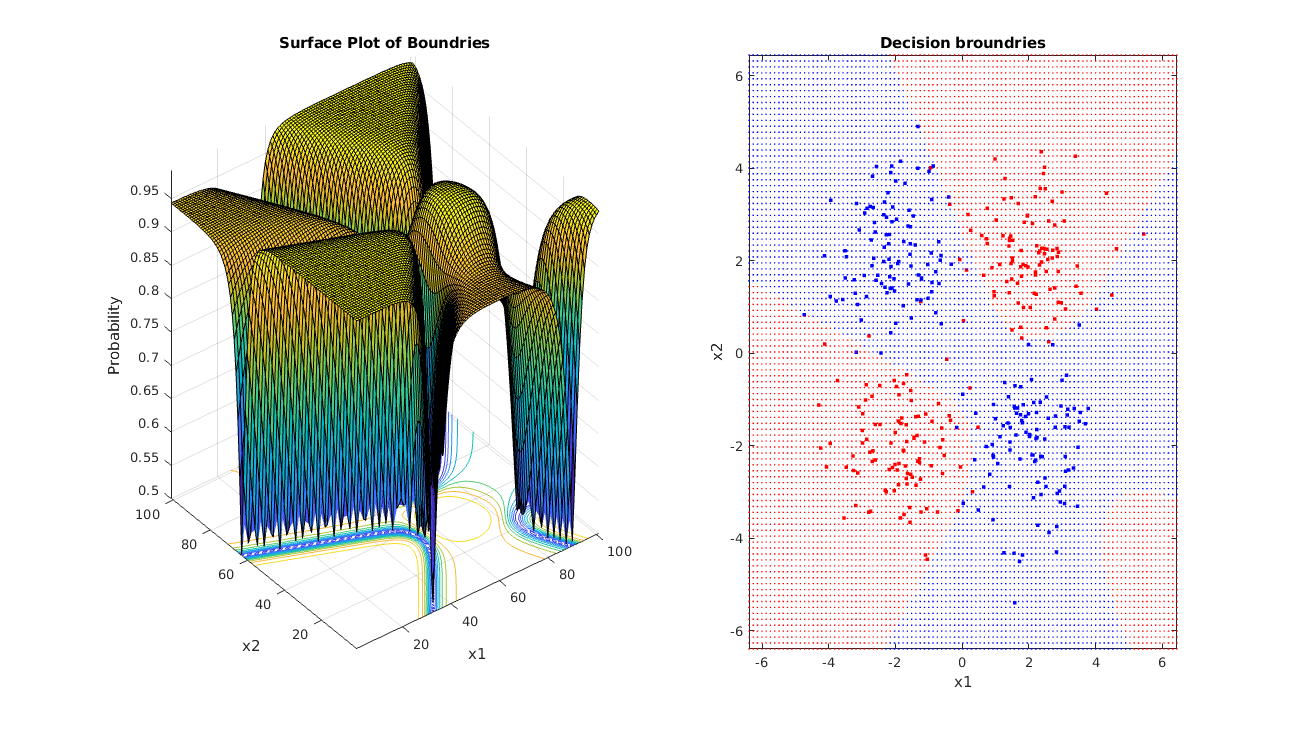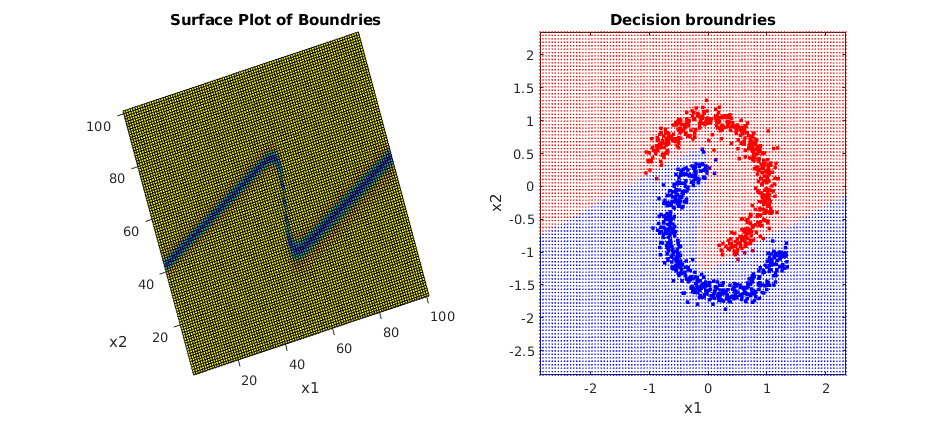## 4. Kernel Learning & regularization

#### Kernal Learning

(Linear, Polynomial, Gaussian)

• Linear
• Polynomial
• Gaussian (RBF)

### Installation::

`pip install regml`

### Execute

``````import regml
regml.GUI()
``````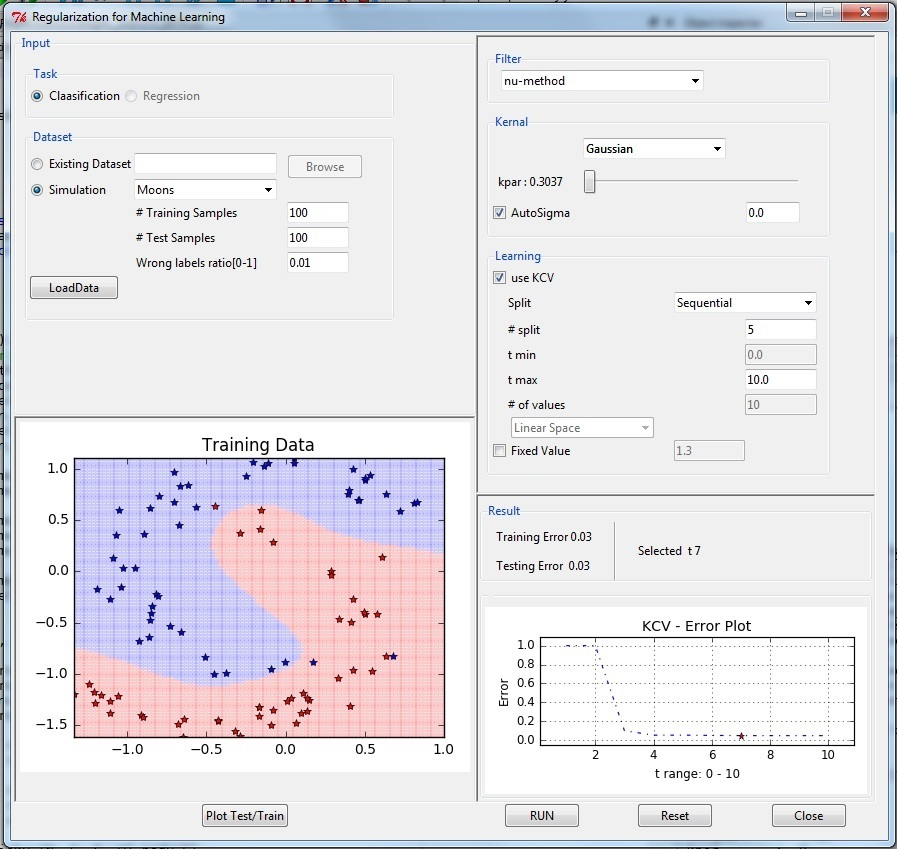## 5 Naive Bayes

### Probabilistic model

Classifier based on Bayes rule: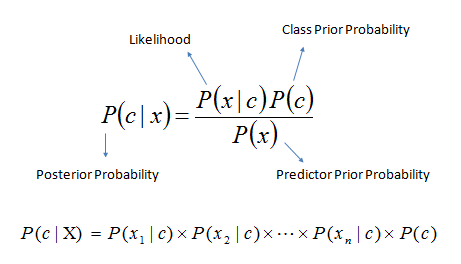### Example with jupyter notebook here and Repository

Notebook include example of Iris data, Breast Cancer and Digit classification (MNIST)

### Notebook

here is code snippet

``````import numpy as np
import matplotlib.pyplot as plt

# For dataset
from sklearn import datasets
from sklearn.model_selection import train_test_split

# Library provided
#from probabilistic import NaiveBayes (NO NEED OF THIS)

##NOW USE spkit library (pip intall spkit)
from spkit.ml import NaiveBayes

X = data.data
y = data.target

Xt,Xs,yt,ys = train_test_split(X,y,test_size=0.3)

print(Xt.shape,yt.shape,Xs.shape,ys.shape)

# Fitting model (estimating the parameters)
clf = NaiveBayes()
clf.fit(Xt,yt)

# Prediction
ytp = clf.predict(Xt)
ysp = clf.predict(Xs)

print('Training Accuracy : ',np.mean(ytp==yt))
print('Testing  Accuracy : ',np.mean(ysp==ys))

print(clf.parameters)

# Visualization
fig = plt.figure(figsize=(12,10))
clf.VizPx()
``````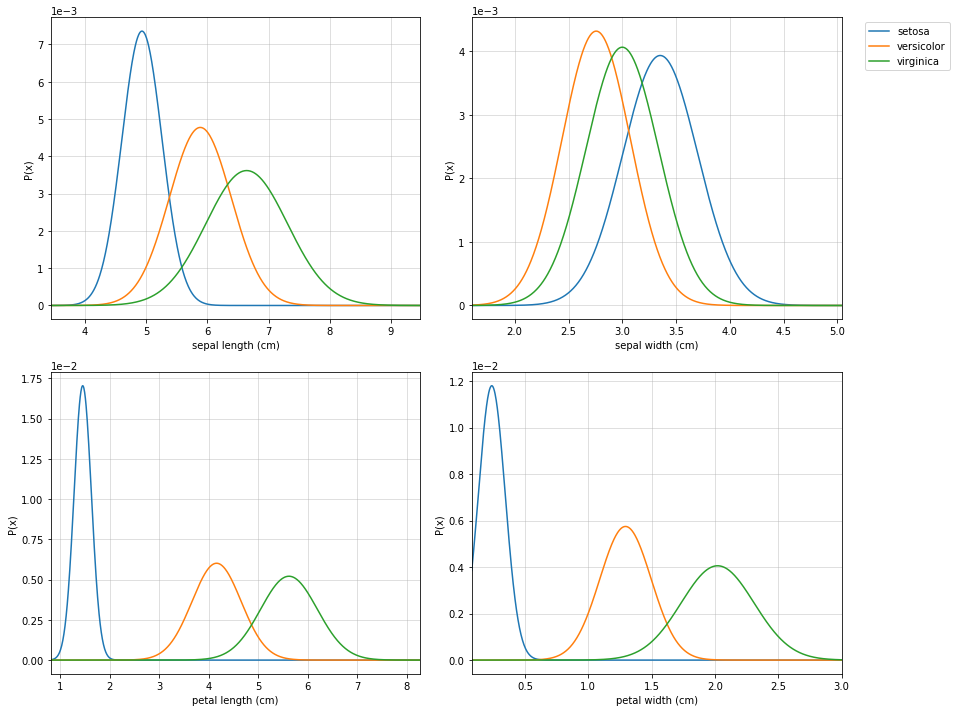## 6 Decision Trees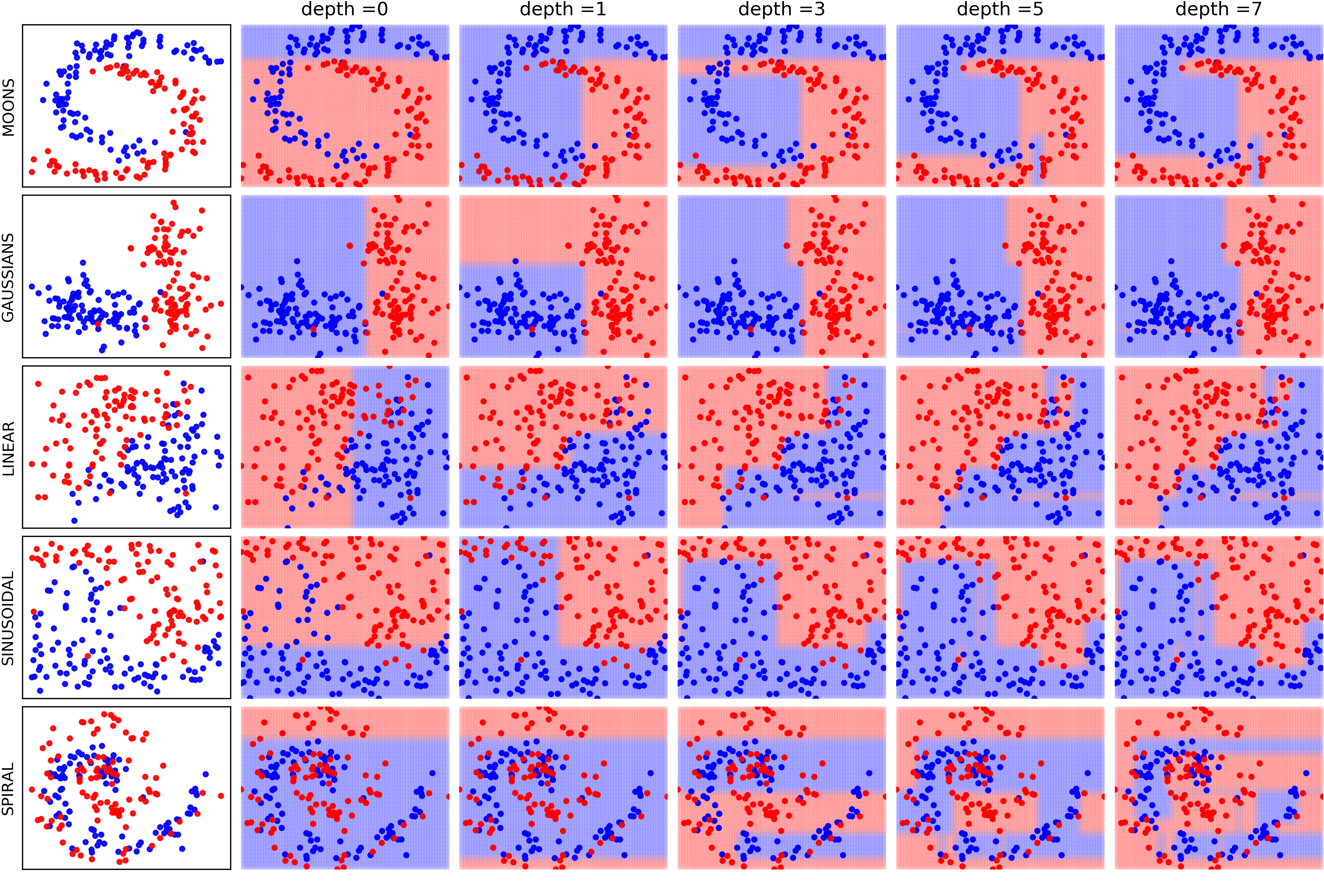### Installation (Now in spkit library)

`pip install spkit`

### Notebook

#### Import

``````import numpy as np
import matplotlib.pyplot as plt

#(NO NEED OF THIS)
#Download trees.py and keep in current directory or give a path (if you know how to)
#from trees import ClassificationTree, RegressionTree

##NOW USE spkit library (pip intall spkit)
from spkit.ml import ClassificationTree, RegressionTree

# For examples
from sklearn import datasets
from sklearn.model_selection import train_test_split
``````

#### Iris Data

``````data = datasets.load_iris()
X = data.data
y = data.target

feature_names = data.feature_names #Optional
Xt,Xs, yt, ys = train_test_split(X,y,test_size=0.3)
``````

#### Initiate the classifier and train it

``````clf = ClassificationTree()

# verbose 0 for no progress, 1 for short and 2 for detailed.
# feature_names is you know, else leave it or set it to None

clf.fit(Xt,yt,verbose=2,feature_names=feature_names)
``````

#### Plot the decision tree

``````# Plot Tree that has been learned
plt.figure(figsize=(15,8))
clf.plotTree(show=True)
``````

### Visualizing the tree building while training

#### Classification: Iris Data, Breast cancer Data Regression::Bostan House price Data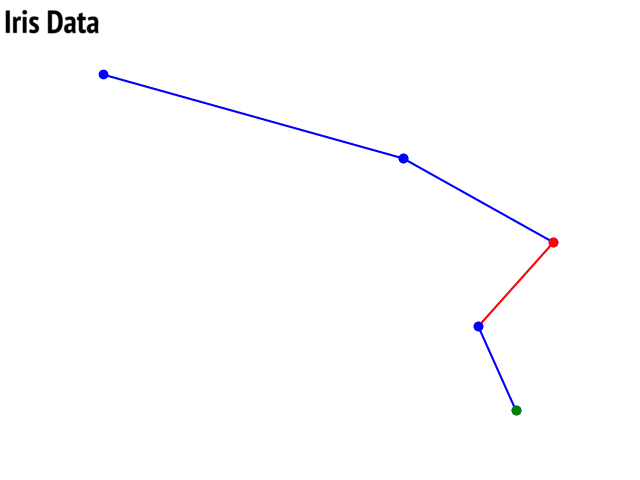### Visualization of decision tree after fitting a model

Option to show colored branch: Blue for True and Red for False Or just show all branches as blue with direction to indicate True and False branch

Iris data: Decesion Tree | Cancer data: Decesion Tree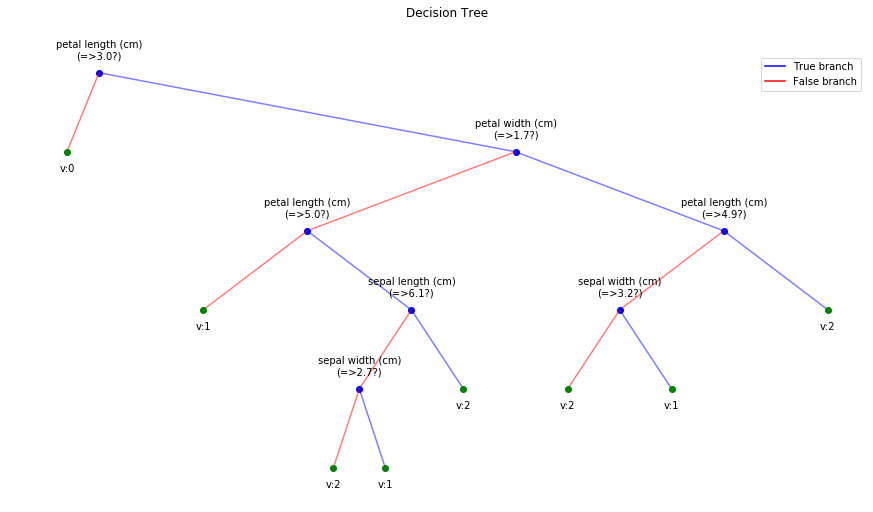|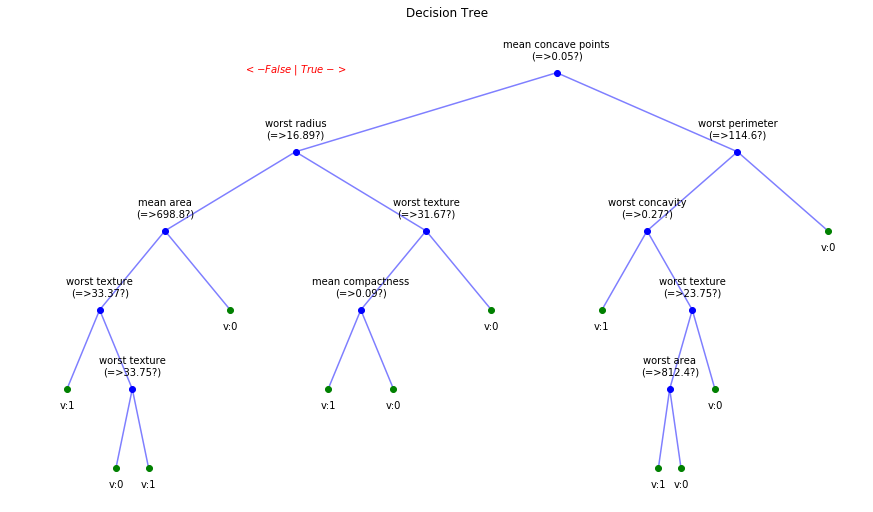Boston data: Decesion Tree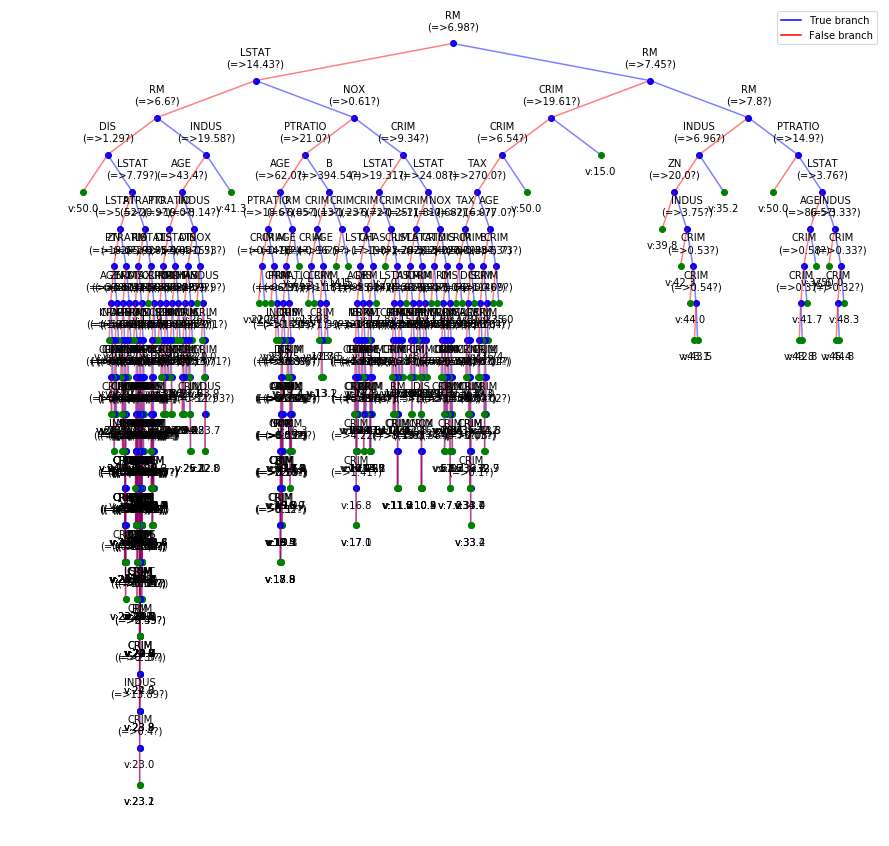### Visualizing the progress of tree building while training

Tree building for Cancer Data (Classification)

Detailed view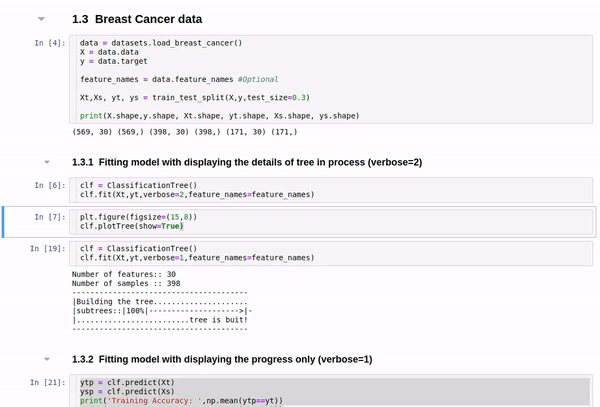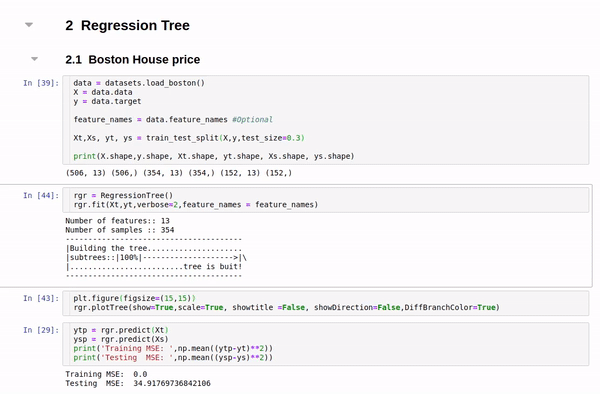Short view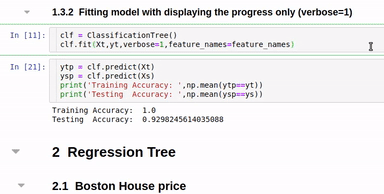Get A Weekly Email With Trending Projects For These Topics
No Spam. Unsubscribe easily at any time.
Python (840,414
Jupyter Notebook (163,805
Learning (75,841
Machine Learning (38,727
Tree (20,382
Neural (16,616
Neural Network (15,797
Visualization (15,440
Classification (13,351
Visualisation (2,728
Logistic Regression (1,605
Decision Trees (1,437
Regularization (1,147
Naive Bayes Classifier (394
Probabilistic Models (107
Kernel Learning (4